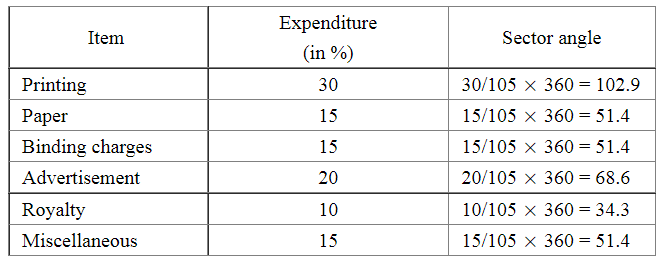# Following data gives the break up of the cost of production of a book:

Question:

Following data gives the break up of the cost of production of a book:

 Printing Paper Binding charges Advertisement Royalty Miscellaneous 30% 15% 15% 20% 10% 15%

Draw a pie- diagram depicting the above information.

Solution:

We know:

Central angle of a component $=\left(\right.$ component value $/$ sum of component values $\left.\times 360^{\circ}\right)$

Here, total expenditures = 105%

Thus, the central angle for each component can be calculated as follows:Total : 105%

Now, the pie chat representing the given data can be constructed by following the steps below:

Step 1 : Draw circle of an appropriate radius.

Step 2 : Draw a vertical radius of the circle drawn in step 1.

Step 3 : Choose the largest central angle. Here the largest central angle is 102.9o. Draw a sector with the central angle 102.9o in such a way that one of its radii coincides with the radius drawn in step 2 and another radius is in its counter clockwise direction.

Step 4 : Construct other sectors representing the other items in the clockwise sense in descending order of magnitudes of their central angles.

Step 5 : Shade the sectors with different colours and label them, as shown as in figure below.​​